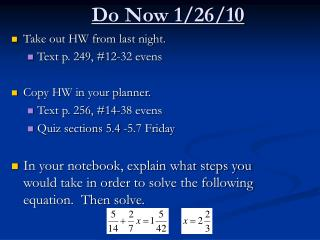DownloadDownload PresentationDo Now 1/26/10

Do Now 1/26/10

Download PresentationDo Now 1/26/10

- - - - - - - - - - - - - - - - - - - - - - - - - - - E N D - - - - - - - - - - - - - - - - - - - - - - - - - - -
Presentation Transcript

1. Do Now 1/26/10 • Take out HW from last night. • Text p. 249, #12-32 evens • Copy HW in your planner. • Text p. 256, #14-38 evens • Quiz sections 5.4 -5.7 Friday • In your notebook, explain what steps you would take in order to solve the following equation. Then solve.

2. 12) 40 14) -48 16) -1 1/3 18) -1 2/5 20) 11 2/3 22) 33 24) -1/4 26) 2/3 28) 31/35 30a) 5/8 square inches 30b) 3/4x 30c) 5/8 + 3/4x = 1; x = 1/2 inch 32a) 1/5 32b) 3750 spectators 32c) 1000 home team fans Homework Text page 249, #12-32 evens

3. Remember this??? To solve equations with fractions, so far you followed these steps: Subtract 4/5 from each side. Write fractions using LCD (1) Undo addition and/or subtraction to get the variable alone on one side of the equation. Multiply each side of the equation by the MULTIPLICATIVE INVERSE of -7/15 (2) Multiply both sides of the equation by the multiplicative inverse of the variable term. -1 Multiply Divide (cancel) common factors 1

4. Objective • SWBAT use the LCD to solve equations and inequalities.

5. Section 5.7, “Equations and Inequalities with Rational Numbers” **Another way to solve an equation with fractions is to clear fractions by multiplying the WHOLE EQUATION by the LCD of the fractions. The resulting equation is equivalent to the original equation WITHOUT fractions.

6. Solving Equations by Clearing Fractions Find LCD of all fractions in the equation, then multiply the whole equation by the LCD. Use distributive property and simplify each term of the equation. Two-step equation. Solve for x.

7. Try It Out!!Solve

8. Solving Equations by Clearing Decimals Look for the greatest number of decimal places. Then multiply the WHOLE EQUATION by that power of 10. Use distributive property and simplify each term of the equation. Two-step equation. Solve for m.

9. Try It Out!!Solve

10. Solving Inequalities by Clearing Fractions Find LCD of all fractions in the inequality, then multiply the whole inequality by the LCD. Use distributive property and simplify each term of the inequality. Two-step inequality. Solve for x. Don’t forget to flip the inequality when dividing by a negative.

11. Try It Out!!Solve

12. Homework • Text p. 256, #14-38 evens

13. 2) 22 4) 30 6) 38 8) 1 3/4 10) -11/24 12) -5/14 14) 35 16) -36 18) 22 20) -1/5 22) -1/6 24) 1/10 26) 80 days Flu Shot: A JAB WELL DONE Fourth of July: JUST UNDER EIGHT DAYS Homework Punchline worksheet #79 and practice worksheet 5.6 Form B evens Practice worksheet 5.6 B evens Punchline worksheet #79